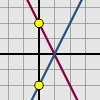#### You may also like### Enclosing Squares

Can you find sets of sloping lines that enclose a square?### Diamond Collector

Collect as many diamonds as you can by drawing three straight lines.### Reflecting Lines

Investigate what happens to the equations of different lines when you reflect them in one of the axes. Try to predict what will happen. Explain your findings.

# Parallel Lines

### Why do this problem?

This resource allows students to explore the connection between a straight line on a graph and the equation that represents or defines it - equations of the form $y = mx + c$. Students are encouraged to conjecture and test their conjectures.

### Possible approach

This problem could be used after How Steep is the Slope?

It is useful if students have also done some preliminary work plotting straight line graphs.

Working with the whole group demonstrate the interactivity, moving both points to allow the group to see the freedom the line has. Draw attention to the equation of the line showing beneath the graph.

Take time to discuss how an equation represents a line by defining the set of points that lie along it. Select points on the line to demonstrate how the coordinates of the point satisfy the equation. Ask questions to challenge this understanding, like: find the equation of a line that goes through (2,3); find the equation of a steeper line; find the equation of a line that slopes down instead of up.

The second interactivity allows the equations of two independent lines to be compared. Working in pairs at computers ask students to propose equations of parallel lines and use the interactivity to check their suggestions.

Encourage students to refine their earlier conjectures. Ask them to phrase what they have learned about equations of straight lines in exactly 25 words.

When students feel they understand this completely, suggest they play Diamond Collector in pairs or against the computer. Allow them to close the game and go back to the parallel lines interactivity if they find they need to learn a bit more to help them play the game better.

### Key questions

What are the connections between the properties of a line and its equation?

What does parallel mean?
How do the equations of parallel lines relate to each other and why is that?
If I give you a rule, can you predict what the graph will look like without having to plot it?

### Possible support

Concentrate on the first interactivity until the relationship between a line's properties and its equation are well understood. Ask students to predict what the graphs of different equations will look like and then use the interactivity to test their predictions.

### Possible extension

Students can extend their understanding by considering the relationship between lines which are perpendicular, establishing the validity of the standard result rather than merely remembering it. This is the task in the related problem Perpendicular Lines

Which is Bigger? offers students the chance to apply their understanding of straight line graphs.